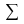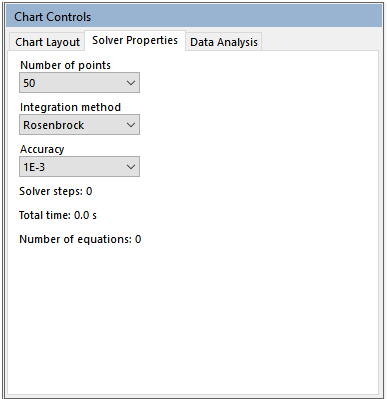Scale-up Suite Help
Set solver properties for simulation
 Simulator > Running models > Set solver properties for simulation

Accessing Solver properties

To view or edit the Solver properties go to the run controls toolbar and click on the Edit Solver icon.Setting Solver Properties in the Excel model process sheet

 Solver Method BE || Options are: BE, Rosen, LSODA Accuracy 1.00E-02 || Allowable range: 1e-2 to 1e-8

With the Solver keyword you can set the accuracy and method for integrating the model.  The abbreviations for the solver methods are:

 BE Backwards Euler Rosen Rosenbrock LSODA LSODA

Editing for number of points to be plotted

The number of points to be plotted affects the level of detail and smoothness of the displayed results. The default number (50) is normally sufficient, but more or less points can be plotted. The actual number of points displayed equals the number of points requested plus any data points plus a few initial points to ensure a smooth startup.

You can select a number of points using the Solver Properties dialog. You can also define the number of points on your process tab:

 Solver Points 120

Changing the integration method (solver type)

Choice of solver algorithms should be made on the basis of simulation run-time. In general, the default routine (Rosenbrock) will be adequate, but occasionally one of the other solver routines will be faster, reducing run times. As a general rule, Backwards Euler is the simplest, so it is recommended to try this solver next with an accuracy of 0.01 if run times with the Rosenbrock solver are too long.

Backwards Euler

In general it will take more steps than the other solvers because it is a lower order solver. The run time of Backwards Euler can increase dramatically if solver accuracy is increased, so should only be used at low accuracies (e.g. 1e-2 or 1e-3). Backwards Euler explicitly prevents any integrated variable becoming negative, which means it can behave better at these lower accuracies.

LSODA

The LSODA solver is a third party ODE solver. It is accurate, but can tend to run slowly for many applications. LSODA actually comprises two solvers, the non-stiff solver Adams-Moulton, and the stiff solver BDF (Backward Differentiation formula). It switches between these two depending on how stiff the problem is. The LSODA solver originates in the netlib repository.

Editing solver accuracy

The solver accuracy can also be set. The default accuracy (0.001) is normally sufficient, but this can be adjusted if higher accuracy is required. Available accuracies range from 0.01 to 1x10-8. Increasing the accuracy will in general increase run-time, but the effect may only be significant with very high accuracies (1x10-6 to 1x10-8).

What are 'Solver steps', 'Total time' and 'Number of Equations'

’Solver steps’, ‘Total time’ and ‘Number of Equations’ are indicators of the solver run. They are updated after each run and can be used to compare solvers/models for run times:

• Solver steps: This reports the total number of steps taken by the solver between time zero and the specified end time. A large number of steps (several thousand) indicates that the problem is difficult to solve and that trying another solver may help. This will be independent of the computer on which the simulation was run.
• Total time: This reports the total time it took to run the simulation. It is closely related to the number of solver steps, i.e. the more steps there are the longer the run time. The value will depend on the computer you are using (processor speed, current level of CPU usage) and can be used to compare different computers in running DynoChem models. The time reported here will be approximately the time it takes to do one run in fitting or optimization.
• Number of Equations: This reports the number of equations that are being solved or integrated. The more equations there are, the slower the simulation is likely to be. Typical DynoChem models have 5 to 10 equations, whereas 20 or more equations may run quite slowly. It may be possible to simplify the model to reduce the number of equations.

Models that run slowly

Show me more on factors that affect run time.

Show me help on troubleshooting slow models.